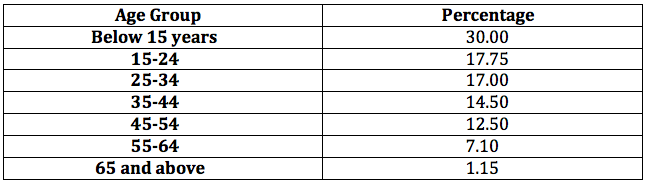Question 142

# The table below shows the age-wise distribution of the population of Reposia. The number of people aged below 35 years is 400 million.If the ratio of females to males in the ‘below 15 years’ age group is 0.96, then what is the number of females (in millions) in that age group?

Solution

64.75% population is below 35 years age.

The number of people aged below 35 years is 400 million.

So total number of people are, suppose x = $$\frac{400*100}{64.75}$$

Now below 15 years of age we have f/m = 0.96 so f = (m+f)*0.96/1.96 and m+f = 30*400/64.75

Solving we get, f = 30*400*0.96/(64.75*1.96) = 90.8

Hence option B .

• All Quant CAT Formulas and shortcuts PDF
• 30+ CAT previous papers with solutions PDF

##### Shobha Meena

2 years, 7 months ago

Isme 1.96 value kaise aayi

##### Hreeshav Roy

2 years, 5 months ago

https://youtu.be/kibVQYr0at0
Hope this helps

OR